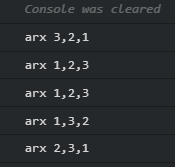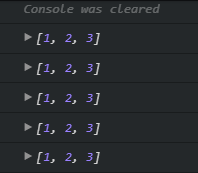# Console Result with some text and without

Console Result with some text and without
0

#1

I was wondering why with some text put in console.log(): (example: console.log(" some " + arr)this function
works as designed and without it only works first time ??
FOR SIMPLICITY, shuffle change the order in a random way --> not exactly random but almostI know that array is a reference type and I only give an address to calculate and I am aware that sort changes the original array.

WITH SOMETHING IN CONSOLE "text " + arrx

``````let arr = [1, 2, 3];

function shuffle(arrx){
arrx.sort(callback);

function callback(a,b){
let x = 0.49 - Math.random();
return x;
}

console.log("arx " + arrx);
}
``````

shuffle(arr);
RESULT(example): arx 1,2,3
shuffle(arr);
RESULT(example): arx 1,2,3
shuffle(arr);
RESULT(example): arx 2,3,1
shuffle(arr);
RESULT(example): arx 2,3,1
shuffle(arr);
RESULT(example): arx 1,3,2

AND WITHOUT TEXT

``````let arr = [1, 2, 3];

function shuffle(arrx){
arrx.sort(callback);

function callback(a,b) {
let x = 0.49 - Math.random();
return x;
}

console.log(arrx);
}
``````

shuffle(arr);
shuffle(arr);
shuffle(arr);
shuffle(arr);
shuffle(arr);
RESULT ALWAYS THE SAME example [1,2,3] or something else multiplied with every shuffle

Regards ;),

#2

I’m assuming you meant

``````console.log(arrx);
``````

In the second example?

They work exactly the same, no difference: result is always random, it makes no difference what is in the console log in addition to the shuffled array. Are sure it’s just not giving you the same result back randomly? For example, I got nine [1,2,3]'s in a row on one run, it doesn’t mean it’s not random

#3

Hmm, I used codesandbox. and it gives me some different results when is text it works normally as expected but only with array it works first time and next results are the same as first one

#4

I’m sorry I make mistake when pasted here

in first one there is console.log("arx " + arrx);

and second function have only

console.log(arrx);

First oneSecond One